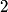## Solution to 1986 Problem 89

 The given wavefunction is symmetric under the exchange operator. This means that if the indices$1$ and$2$ are switched on the right-hand side, then the resulting wavefunction is not changed. A particle has a symmetric wavefunction if and only if it is a boson i.e. if and only if it has integral spin. The deuteron consists of a proton and a neutron, each of which have spin 1/2. According to Griffiths QM equation 4.184, the possible spin quantum numbers for the deuteron are then 0 or 1, meaning that the deuteron is a boson. Therefore, the correct answer is (E).